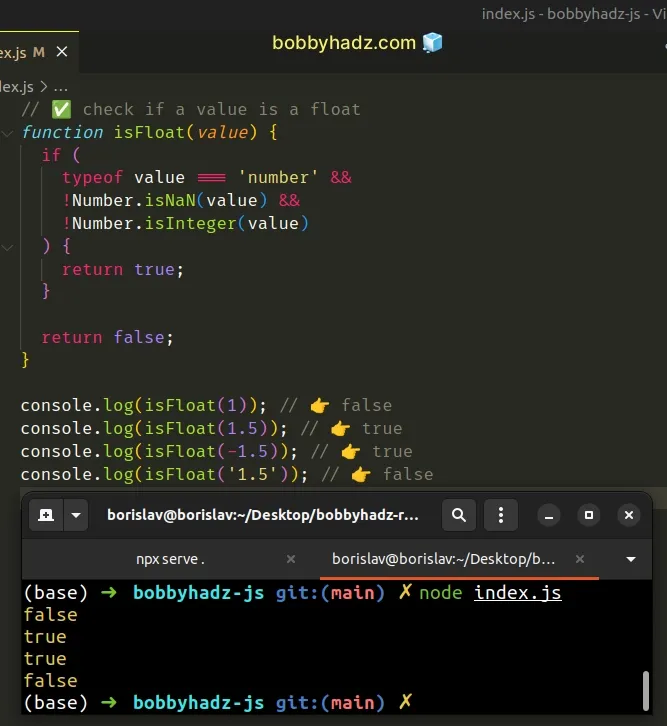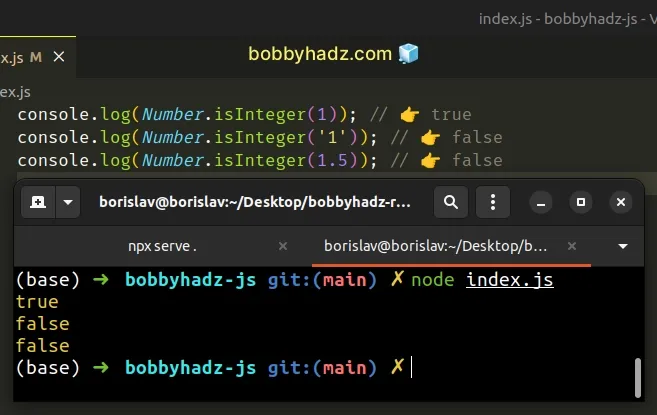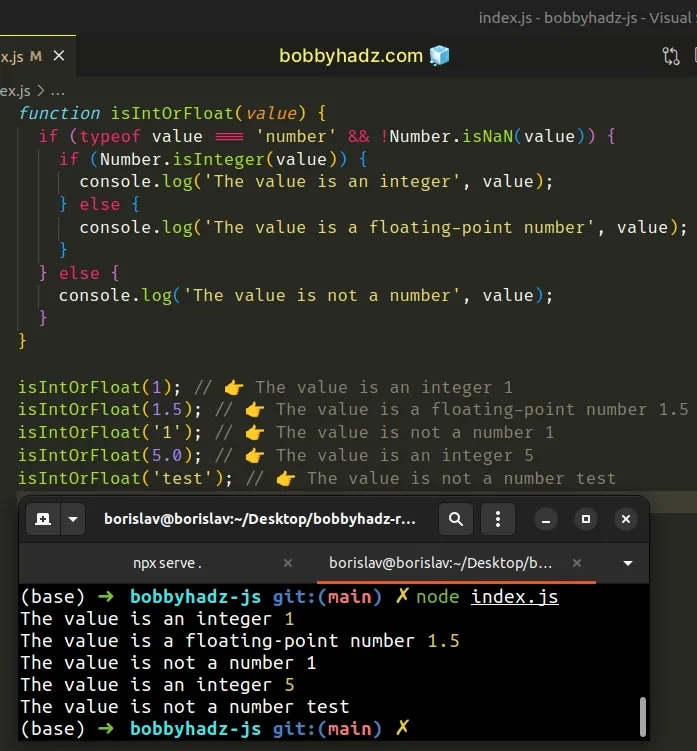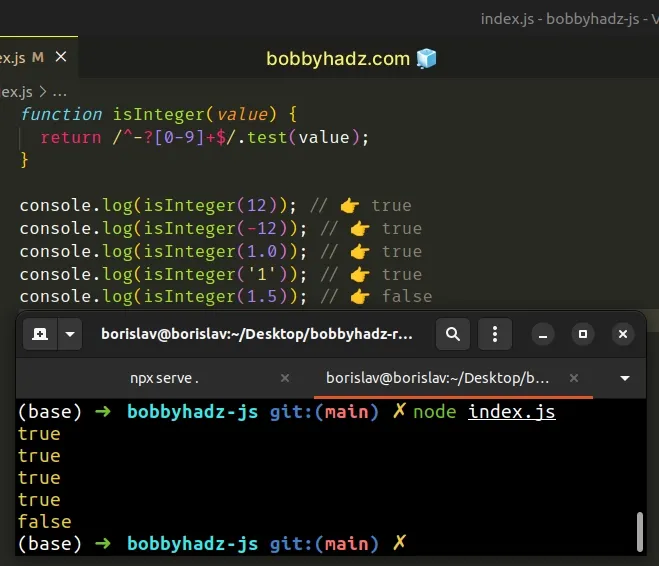# Check if a value is a Float or an Integer in JavaScriptLast updated: Dec 27, 2022
4 min## #Check if a value is a Float or an Integer in JavaScript

To check if a value is a float or an integer:

1. Use the `Number.isInteger()` method to check if a value is an integer.
2. Check if the value has a type of number and is not an integer or `NaN` to check if the value is a float.
index.js
```Copied!```// ✅ check if a value is a float
function isFloat(value) {
if (
typeof value === 'number' &&
!Number.isNaN(value) &&
!Number.isInteger(value)
) {
return true;
}

return false;
}

console.log(isFloat(1)); // 👉️ false
console.log(isFloat(1.5)); // 👉️ true
console.log(isFloat(-1.5)); // 👉️ true
console.log(isFloat('1.5')); // 👉️ false
``````To check if a number is a float, check if the value has a type of number and is not an integer or `NaN`.

We first check if the provided value has a type of number. If it doesn't we return `false` straight away.

index.js
```Copied!```function isFloat(value) {
if (
typeof value === 'number' &&
!Number.isNaN(value) &&
!Number.isInteger(value)
) {
return true;
}

return false;
}
``````

We used the logical AND (&&) operator to check for multiple conditions. For our `if` block to run, all conditions have to be met.

The second condition checks if a value isn't `NaN` (not a number). Unfortunately, `NaN` has a type of `number` in JavaScript.

index.js
```Copied!```console.log(typeof Number.NaN); // 👉️ number
``````

If the provided value is of type `number`, is not `NaN` and is not an integer, then the value is a floating-point number.

## #Checking if a value is an integer

We used the `Number.isInteger()` method to check if a value is an integer.

index.js
```Copied!```console.log(Number.isInteger(1)); // 👉️ true
console.log(Number.isInteger('1')); // 👉️ false
console.log(Number.isInteger(1.5)); // 👉️ false
``````There is a catch when using the Number.isInteger() method.

It returns `true` if the passed-in value:

• is an integer
• is a float that can be represented as an integer

Here's an example of a float that can be represented as an integer.

index.js
```Copied!```console.log(Number.isInteger(10.0)); // 👉️ true
``````

It depends on your use case whether you consider `10.0` to be an integer or float.

Our implementation of the function considers numbers like `1.0` and `5.0` to be integers.

## #A custom function that checks if the value is int or float

You can combine the conditions into a single function to check if a value is a float or an integer.

index.js
```Copied!```function isIntOrFloat(value) {
if (typeof value === 'number' && !Number.isNaN(value)) {
if (Number.isInteger(value)) {
console.log('The value is an integer', value);
} else {
console.log('The value is a floating-point number', value);
}
} else {
console.log('The value is not a number', value);
}
}

isIntOrFloat(1); // 👉️ The value is an integer 1
isIntOrFloat(1.5); // 👉️ The value is a floating-point number 1.5
isIntOrFloat('1'); // 👉️ The value is not a number 1
isIntOrFloat(5.0); // 👉️ The value is an integer 5
isIntOrFloat('test'); // 👉️ The value is not a number test
``````The function first checks if the value is a number and is not `NaN` because `NaN` has a type of `number` in JavaScript.

If we enter the `if` block, we know that the value is a number.

The next step is to check if the value is an integer using the `Number.isInteger()` function.

If the value is an `integer`, the `if` block runs.

If the value is a number and is not an integer, then the value is a floating-point number and the `else` block runs.

If the initial conditional check fails and the value does not have a type of `number` or is `NaN`, then the value is not a number.

## #Checking if the value is a valid integer using `RegExp.test()`

You can also use the `RegExp.test()` method to check if a value is a valid integer.

index.js
```Copied!```function isInteger(value) {
return /^-?[0-9]+\$/.test(value);
}

console.log(isInteger(12)); // 👉️ true
console.log(isInteger(-12)); // 👉️ true
console.log(isInteger(1.0)); // 👉️ true
console.log(isInteger('1')); // 👉️ true
console.log(isInteger(1.5)); // 👉️ false
``````The RegExp.test method matches the specified regular expression against the supplied value and returns `true` if the regular expression is matched in the string and `false` otherwise.

The forward slashes `/ /` mark the beginning and end of the regular expression.

The caret `^` matches the beginning of the input and the dollar sign `\$` matches the end of the input.

We used a hyphen to also allow for negative integers.

The question mark `?` matches the preceding item (the minus) 0 or 1 times. In other words, the minus `-` might be there, or it might not be there.

The character class `[0-9]` matches the digits in the range.

The plus `+` matches the preceding item (the range of digits) one or more times.

If you ever need help reading a regular expression, check out this regular expression cheatsheet by MDN.

It contains a table with the name and the meaning of each special character with examples.